# Effect of the speed of the algorithm's convergence on the quality of distributed computing in WSN

Autor: M. Kenyeres, J. Kenyeres, V. Škorpil <kenyeres(at)phd.feec.vutbr.cz>, Pracoviště: Vysoké učení technické v Brně, Téma: Optimalizace, Vydáno dne: 20. 04. 2015

The paper presents the results of the practical experiments whose goal is to show the effect of changing the parameter the speed of the algorithm's convergence on the parameters average deviation, average percentage deviation (both parameters are for expressing the algorithm's precission) and the number of iterations necessary for each node to achieve a consensus. We also described the main features of wireless sensor networks and distributed computing in these networks.

Keywords: WSN; the speed of the convergence; distributed computing

### Wireless sensor networks

Wireless sensor networks (WSN) are defined as networks formed by distributed independent devices called nodes deployed in a particular geographical area. The purpose of the nodes is to monitor a particular physical quantity. Consequently, this measured value is supposed to be processed and sent to other nodes via a wireless transmission medium. These procedures allow WSN to work as a distributed system fulfilling a particular task. The typical architecture of a node is depicted in the Figure 1: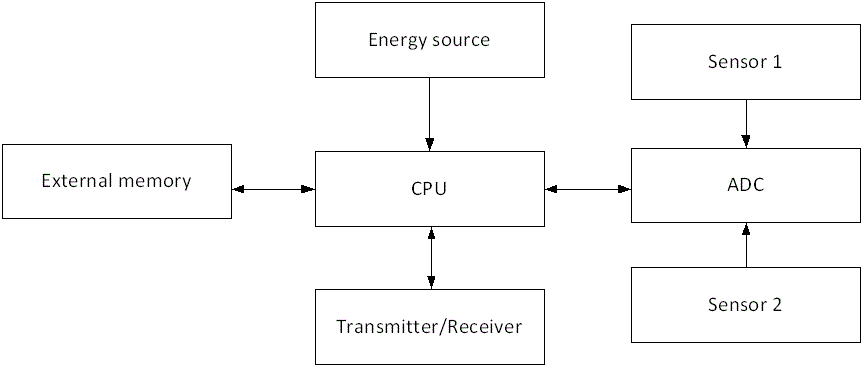Fig. 1 The architecture of a node

A node is formed by a transmitter and receiver, a microcontroller and an energy source. The microcontroller works as a controlling element responsible for data processing, analog-to-digital conversion of a measured value and controlling the other parts forming a node. Nodes features are supposed to involve the following: small energy consumption, programmability and the ability to work in a low-energy mode. The goal of the transmitter and receiver is to send and receive messages in the frequency bandwidth 433 MHz – 2,4 GHz. Usually a battery or a solar cell can be used as an energy source. The sensor is a hardware device to measure a particular physical value.

### Distributed computing

The distributed algorithms’ functionality relates to parallel distributed systems formed by separated subparts whose correlative communication is very limited (,). In spite of the mentioned fact, these subparts are able to cooperate with each other in order to fulfil particular goals. In this publication, we implemented the Average consensus algorithm into WSN, utilizing the recommendations about calibration of the convergence event presented in (). We assume that each node forming a wireless sensor network is able to achieve the average value calculated from all the nodes’ initial values in a distributed manner just according to messages sent by adjacent neighbours and inner states from the previous iteration (,). According to obtained knowledge from (,,), we described WSN using the graph theory tools. We consider a network to be an indirect graph defined as follows: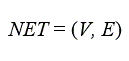(1)

NET is the label of a graph which consists of the set V and E. V is a set of all the vertexes presented in the graph V = {v1, v2....vN}.. The parameter N determines the number of vertexes and so, a wireless sensor network’s size. Nodes connected to each other are represented by vertexes between which an edge exists. The mentioned connection is named as a path ei,j. E forms a subset of the Cartesian multiplication EVxV. Since we consider an indirect graph, ei,j implies the existence of ej,i. The Average consensus algorithm, which we implemented into a wireless sensor network, is a distributed algorithm based on the idea that each node converges to the average value in a distributed manner etc. just very limited information is available for nodes. Each node converges to the value determined as follows: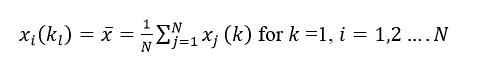(2)

Here, the indexes i (vi∈V) and j (vj∈V)are the indexes of the corresponding vertexes. The parameter k is the number of an iteration and its index l indicates the last iterations and therefore, also the number of iterations necessary for a network to achieve the consensus. The vector x∈RN is a variable regarding to the time and is formed by nodes’ inner values. As mentioned, each node converges to the average value in every iteration, which can be described as follows:(3)

A∈{0,1}NxN is the adjacent matrix (,) whose goal is to describe neighbours’ relations between particular nodes. The function values of A are defined according to the following rules: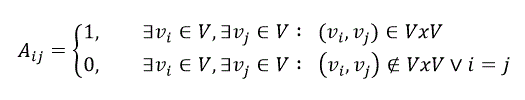(4)

The algorithm is executed in such a way that each node is assigned an initial value which is derived from the identity number assigned to it at the beginning of the process. The current state is calculated just from the inner state and messages sent by adjacent neighbours. The parameter ε is the speed of the algorithm's convergence () and its range of the convergence is determined as follows: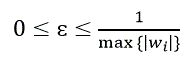(5)

The parameter w is the weight of a node and presents the degree of a vertex. For the node whose index is i, it is defined as follows: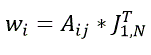(6)

Using mathematical formula, the convergence process may be described as follows ():(7)

Fulfilling the condition (7) is not possible and so, the convergence event to indicate completed tasks has been defined. In the experiment described in this paper, we used the convergence event from . It is proposed so that each node is able to recognize the convergence event in a distributive manner. When the consensus is reached at a particular node, this node does not upgrade its inner state in the next iterations.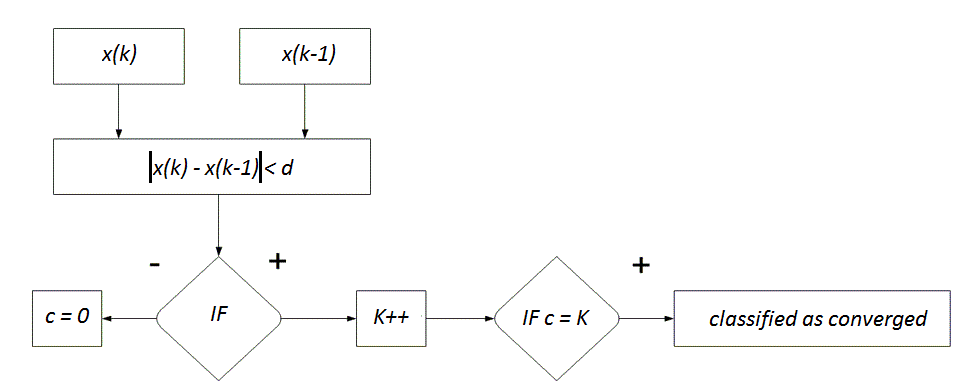Fig. 2 The algorithm determining the convergence event

According to recommendation from , we set the parameter d to the value of 0.0015 and the K parameter to 15. We do it so because the algorithm is supposed to reach a high quality results. In the practical part, we focused on examining effects of ε's changes on the parameters‘s changes on the parameters kl, AD and APD. The mentioned parameters are defined as follows: The definition of AD(average deviation):(8)

This parameter determines average deviation of the final values from the expected calculated according to (2). The definition of ADP(average percentage deviation):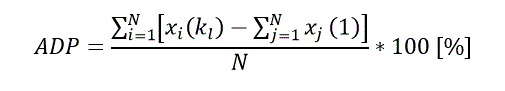(9)

### Experiment

In this paper, we implemented Average consensus algorithm into ten networks containing 30 nodes and whose topologies were dense. The parameter Average Deviation AD was counted as the average calculated from the results obtained in 10 networks. We can see from the results that the higher the parameter ε is, the smaller deviation is observed. From ε = 0.02, the deviation is negligible. At the end, we depicted the maximal and minimal values within obtained results. The parameter variance represents how these obtained results differed from one another. We can see from the results that the variance remains small; therefore, the algorithm’s behaviour is similar despite of the different topologies.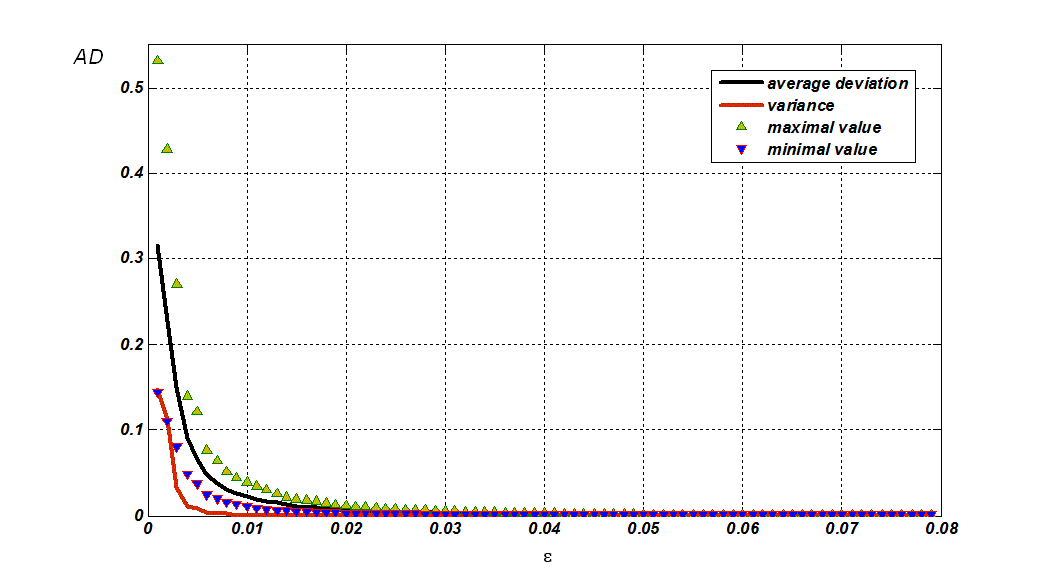Fig. 3 The resulsts of AD

In the next graph, we depicted APD parameter and can observe that the maximal deviation in percentage was 2 percent, which can be classified as a high precision result. The function’s behaviour is similar to the previous graph, where the function decreased as ε grown. We also showed both the maximal and minimal value.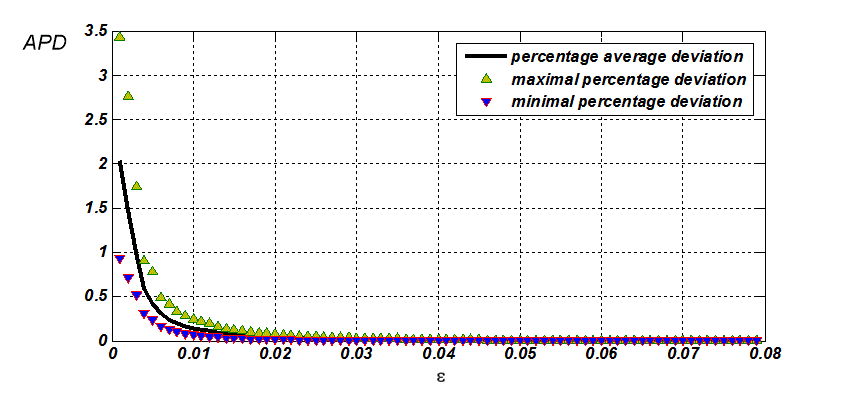Fig. 4 The resulsts of APD

In the last experiment, we examined the effect of ε on the number of iterations necessary for a network to convergence kl. We can see that the function has again a decreasing behavior like in the previous two cases.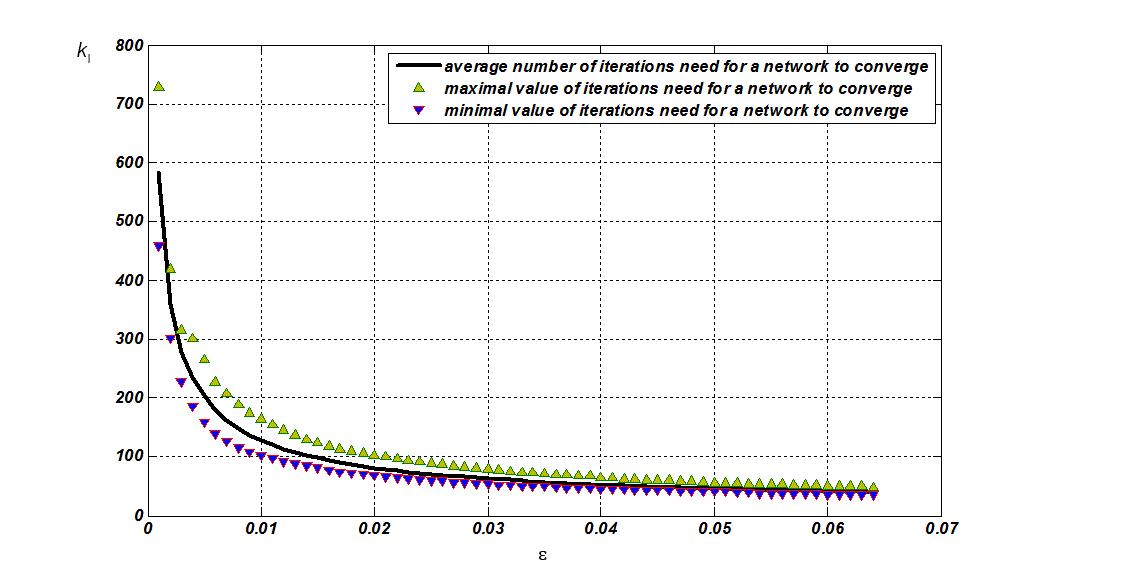Fig. 5 The resulsts of kl

### Contribution

The article presents the results describing how the parameter the speed of the algorithm's convergence ε affects the parameters AD, ADP and kl. We used a distributed convergence event whose parameters d and K were set according to the recommendations from . From the results, we can see that the growth of ε results in the fucntions decreasing.

### Literatura

 Coulouris, G. F., Dollimore, J., & Kindberg, T. (2005). Distributed systems: concepts and design. pearson education.
 Tanenbaum, A., & Van Steen, M. (2007). Distributed systems. Pearson Prentice Hall.
 Kenyeres, M. (2015). Optimalization of Distributed Classification of the Convergence Event
 Kenyeres, J., Kenyeres, M., Rupp, M., & Farkas, P. (2011, April). WSN implementation of the average consensus algorithm. In Wireless Conference 2011-Sustainable Wireless Technologies (European Wireless), 11th European (pp. 1-8). VDE.
 Kenyeres, J., Kenyeres, M., & Rupp, M. (2011, June). Experimental node failure analysis in WSNs. In Systems, Signals and Image Processing (IWSSIP), 2011 18th International Conference on (pp. 1-5). IEEE.
 Biggs, Norman. Algebraic graph theory. Cambridge university press, 1993.
 Andrásfai, Béla. Graph theory: flows, matrices. CRC Press, 1991.
 Foulds, Leslie R. Graph theory applications. Springer Science & Business Media, 1992.
 Gibbons, Alan. Algorithmic graph theory. Cambridge UniversityPress, 1985.
 Bapat, Ravindra B. Graphs and matrices. New York (NY): Springer, 2010.
 Xiao, Lin, Stephen Boyd, and Seung-Jean Kim. "Distributed average consensus with least-mean-square deviation." Journal of Parallel and Distributed Computing 67.1 (2007): 33-46.95, No. 1, pp. 215-233, Jan. 2007.